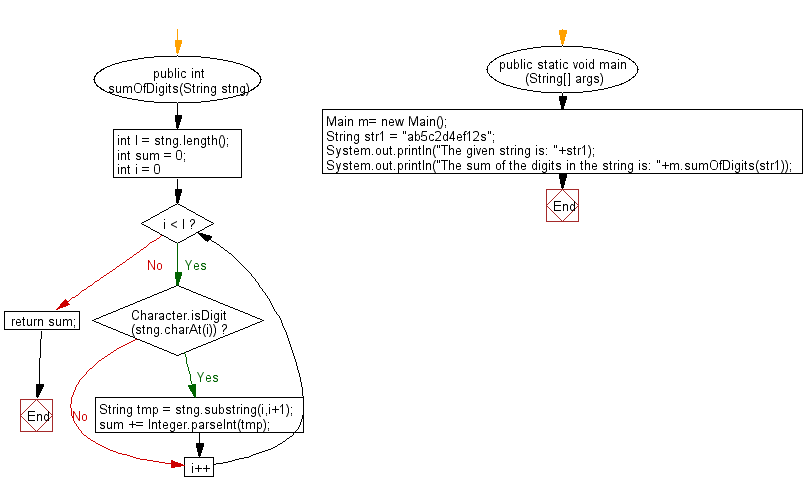﻿ Java exercises: Return the sum of the digits present in the given string - w3resource# Java String Exercises: Return the sum of the digits present in the given string

## Java String: Exercise-95 with Solution

Write a Java program to return the sum of the digits present in the given string. If there is no digits the sum return is 0.

Sample Solution:

Java Code:

``````import java.util.*;
public class Main
{
public int sumOfDigits(String stng)
{
int l = stng.length();
int sum = 0;
for (int i = 0; i < l; i++)
{
if (Character.isDigit(stng.charAt(i)))
{
String tmp = stng.substring(i,i+1);
sum += Integer.parseInt(tmp);
}
}
return sum;
}

public static void main (String[] args)
{
Main m= new Main();
String str1 =  "ab5c2d4ef12s";
System.out.println("The given string is: "+str1);
System.out.println("The sum of the digits in the string is: "+m.sumOfDigits(str1));
}
}
``````

Sample Output:

```The given string is: ab5c2d4ef12s
The sum of the digits in the string is: 14
```

Pictorial Presentation:Flowchart:Java Code Editor:

Improve this sample solution and post your code through Disqus

What is the difficulty level of this exercise?

﻿

## Java: Tips of the Day

Array vs ArrayLists:

The main difference between these two is that an Array is of fixed size so once you have created an Array you cannot change it but the ArrayList is not of fixed size. You can create instances of ArrayLists without specifying its size. So if you create such instances of an ArrayList without specifying its size Java will create an instance of an ArrayList of default size.

Once an ArrayList is full it re-sizes itself. In fact, an ArrayList is internally supported by an array. So when an ArrayList is resized it will slow down its performance a bit as the contents of the old Array must be copied to a new Array.

At the same time, it's compulsory to specify the size of an Array directly or indirectly while creating it. And also Arrays can store both primitives and objects while ArrayLists only can store objects.

Ref: https://bit.ly/3o8L2KH